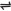## Equilibrium Law and Equilibrium Constant

The law of chemical equilibrium states that in a system at chemical equilibrium, the concentration of the materials which are involved in the reaction must satisfy the condition expressed by the constancy of the Mass Action Expression.

For the hypothetical reaction aA + bBcC + dD, the Mass Action expression at equilibrium is given by;

kf / kb = [C]c [D]d / [A]a [B]b

[ ] represents Concentration in mol/dm3 .

The ratio of the two rate constants gives the equilibrium constant, K.

That is, kf / kb = K

Therefore, Kc = [C]c [D]d / [A]a [B]b

Kc - equilibrium constant for the forward reaction (in terms of concentrations in mol/dm3)

Notice that equilibrium constants expressions for reactions are always for the forward reactions, except when indicated otherwise. For the reverse reaction, equilibrium constant, Kc is

Kc = [A]a [B]b / [C]c [D]d

The product of both equilibrium constants (i.e., the forward and backward reactions) is one.

[C]c [D]d / [A]a [B]b x [A]a [B]b / [C]c [D] = 1

The equilibrium constant of a system can also be expressed in terms of partial pressure, P

Kp = [PC]c [PD]d / [PA]a [PB]b

That is, , Kp represents equilibrium constant in terms of partial pressure. The equilibrium constant, K is related to the free energy of a system by the expression:

∆ G = - RTInK

Note: When the forward reaction is favored, the numerator of the Mass Action Expression becomes greater than the denominator, this causes K to increase (i.e. more than 1) and ∆G = -ve .

When the backward reaction is favored, the denominator of the Mass Action Expression becomes greater than the numerator, this causes K to decrease (i.e. less than 1) and ∆G = +ve.

When both the forward and backward reactions are equal, the numerator of the Mass Action Expression becomes equal to the denominator, this causes K to be 1 and ∆G = 0.  The system is at equilibrium.

Related Posts

 Like This Post? Please Share!!!!!!!

Copyright , All Rights Reserved Free Chemistry Online | About Us | Usage of Content | Total Disclosures | Privacy Policy